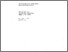# Convergence of Functions: Equi-Semicontinuity

Dolecki, S., Salinetti, G., & Wets, R.J.-B. (1980). Convergence of Functions: Equi-Semicontinuity. IIASA Working Paper. IIASA, Laxenburg, Austria: WP-80-185Preview
Text
WP-80-185.pdf

## Abstract

The ever increasing complexity of the systems to be modeled and analyzed, taxes the existing mathematical and numerical techniques far beyond our present day capabilities. By their intrinsic nature, some problems are so difficult to solve that at best we may hope to find a solution to an approximation of the original problem. Stochastic optimization problems, except in a few special cases, are typical examples of this class.

This however raises the question of what is a valid "approximate" to the original problem. The design of the approximation , must be such that (i) the solution to the approximate provides approximate solutions to the original problem and (ii) a refinement of the approximation yields a better approximate solution. The classical techniques for approximating functions are of little use in this setting. In fact very simple examples show that classical approximation techniques dramatically fail in meeting the objectives laid out above.

What is needed, at least at a theoretical level, is to design the approximates to the original problem in such a way that they satisfy an epi-convergence criterion. The convergence of the functions defining the problem is to be replaced by the convergence of the sets defined by these functions. That type of convergence has many properties but for our purpose the main one is that it implies the convergence of the (optimal) solutions.

This article is devoted to the relationship between the epi-convergence and the classical notion of pointwise-convergence. A strong semicontinuity condition is introduced and it is shown to be the link between these two types of convergences. It provides a number of useful criteria which can be used in the design of approximates to difficult problems.

Item Type: Monograph (IIASA Working Paper) System and Decision Sciences - Core (SDS) IIASA Import 15 Jan 2016 01:47 27 Aug 2021 17:09 http://pure.iiasa.ac.at/1284View Item